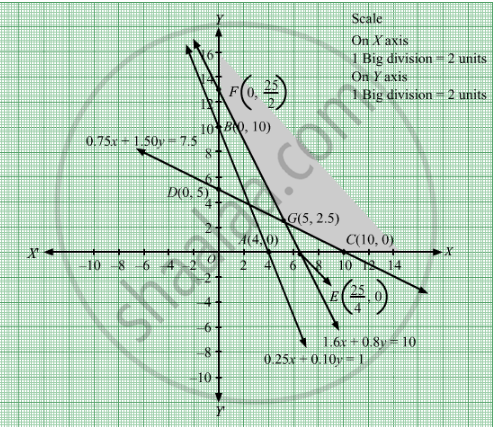# A Diet of Two Foods F1 And F2 Contains Nutrients Thiamine, Phosphorous and Iron. the Amount of Each Nutrient in Each of the Food (In Milligrams per 25 Gms) is Given in the Following Table: - Mathematics

Sum

A diet of two foods F1 and F2 contains nutrients thiamine, phosphorous and iron. The amount of each nutrient in each of the food (in milligrams per 25 gms) is given in the following table:

 Nutrients Food F1 F2 Thiamine 0.25 0.10 Phosphorous 0.75 1.50 Iron 1.60 0.80

The minimum requirement of the nutrients in the diet are 1.00 mg of thiamine, 7.50 mg of phosphorous and 10.00 mg of iron. The cost of F1 is 20 paise per 25 gms while the cost of F2 is 15 paise per 25 gms. Find the minimum cost of diet.

#### Solution

Let 25x grams of food F1 and 25y grams of food F2 be used to fulfil the minimum requirement of thiamine, phosphorus and iron.
As, we are given

 Nutrients Food F1 F2 Thiamine 0.25 0.10 Phosphorous 0.75 1.50 Iron 1.60 0.80

And the minimum requirement of the nutrients in the diet are 1.00 mg of thiamine, 7.50 mg of phosphorous and 10.00 mg of iron.$\text{ Therefore, }$
$0 . 25x + 0 . 10y \geq 1$
$0 . 75x + 1 . 50y \geq 7 . 5$
$1 . 6x + 0 . 8y \geq 10$
$\text{ Since, the quantity cannot be negative }$
$\ ∴ x, y \geq 0$

The cost of F1 is 20 paise per 25 gms while the cost of F2 is 15 paise per 25 gms.Therefore, the cost of 25x grams of food F1 and 25y grams of food F2 is
Rs (0.20x + 0.15y).
Hence,
Minimize Z = $0 . 20x + 0 . 15y$
subject to

$0 . 25x + 0 . 10y \geq 1$
$0 . 75x + 1 . 50y \geq 7 . 5$
$1 . 6x + 0 . 8y \geq 10$
$x, y \geq 0$

First, we will convert the given inequations into equations, we obtain the following equations:
0.25x + 0.10y = 1, 0.75x + 1.50y = 7.51.6x + 0.8y = 10, x = 0 and y = 0.
The line 0.25x + 0.10y = 1 meets the coordinate axis at

A(4,0) and B(0, 10). Join these points to obtain the line 0.25x + 0.10y = 1.
Clearly, (0, 0) does not satisfies the inequation 0.25x + 0.10y ≥ 1. So, the region in xy-plane that does not contains the origin represents the solution set of the given equation.

The line 0.75x + 1.50y = 7.5. meets the coordinate axis at C(10, 0) and D(0, 5). Join these points to obtain the line 0.75x + 1.50y = 7.5.
Clearly, (0, 0) does not satisfies the inequation 0.75x + 1.50y ≥ 0.75. So, the region in xy-plane that does not contains the origin represents the solution set of the given equation.

The line 1.6x + 0.8y = 10 meets the coordinate axis at

$E\left( \frac{25}{4}, 0 \right)$ and $F\left( 0, \frac{25}{2} \right)$ Join these points to obtain the line 1.6x + 0.8y = 10.
Clearly, (0, 0) does not satisfies the inequation 1.6x + 0.8y ≥ 10. So, the region in xy-plane that does not contains the origin represents the solution set of the given equation.

Region represented by x ≥ 0 and y ≥ 0:
Since, every point in the first quadrant satisfies these inequations. So, the first quadrant is the region represented by the inequations.
These lines are drawn using a suitable scale.The corner points of the feasible region are F(0, 12.5), G(5, 2.5), C(10, 0)

The value of the objective function at these points are given by the following table

 Points Value of Z F 0.20(0)+0.15(12.5) = 1.875 G 0.20(5)+0.15(2.5) = 1.375 C 0.20(10) + 0.15(0) = 200

Thus, the minimum cost is at G which is Rs 1.375

Concept: Graphical Method of Solving Linear Programming Problems
Is there an error in this question or solution?

#### APPEARS IN

RD Sharma Class 12 Maths
Chapter 30 Linear programming
Exercise 30.3 | Q 1 | Page 38